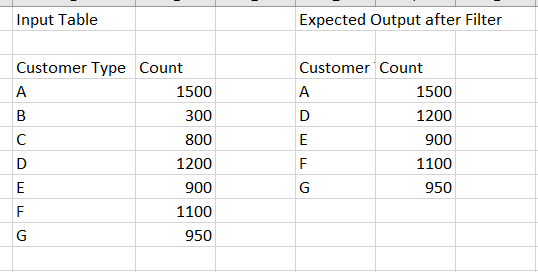# how can we filter the rows whose column1 contains top 80% of count.

I have a table that has a column that contains the count of customers.
I need to filter the Rows that have the top 80% of the Count.I can’t really make sense of your output.
Why would customer types B and C be filtered out but not E?

The total of the counts in your input table is 6750, so 80% is would be 5400.
The total of the counts in your put table is 5650.
How did you decide whether or not to include E?

A general approach that you can explore is:

1. Sort the Count column in descending order
2. Use the Math Formula node to calculate 80% of the sum of the count column
3. Use the Moving Aggregation node to calculate the sum of the count column, making sure that the cumulative computation option is checked.
4. Use the Rule-Based Row Filter node to remove rows where the Cumulative Sum is less than or equal to the value you calculated in step 2
5 Likes

@elsamuel The output table doesn’t have B&C because to get it first we need to sort it in the decreasing order then we have to pick the top rows whose count have atleast 80%.

@PankajChaudhary , this workflow is based on @elsamuel 's suggestion, and takes into account your rule clarification.

Filter top 80 percent.knwf (25.3 KB)2 Likes

This topic was automatically closed 7 days after the last reply. New replies are no longer allowed.